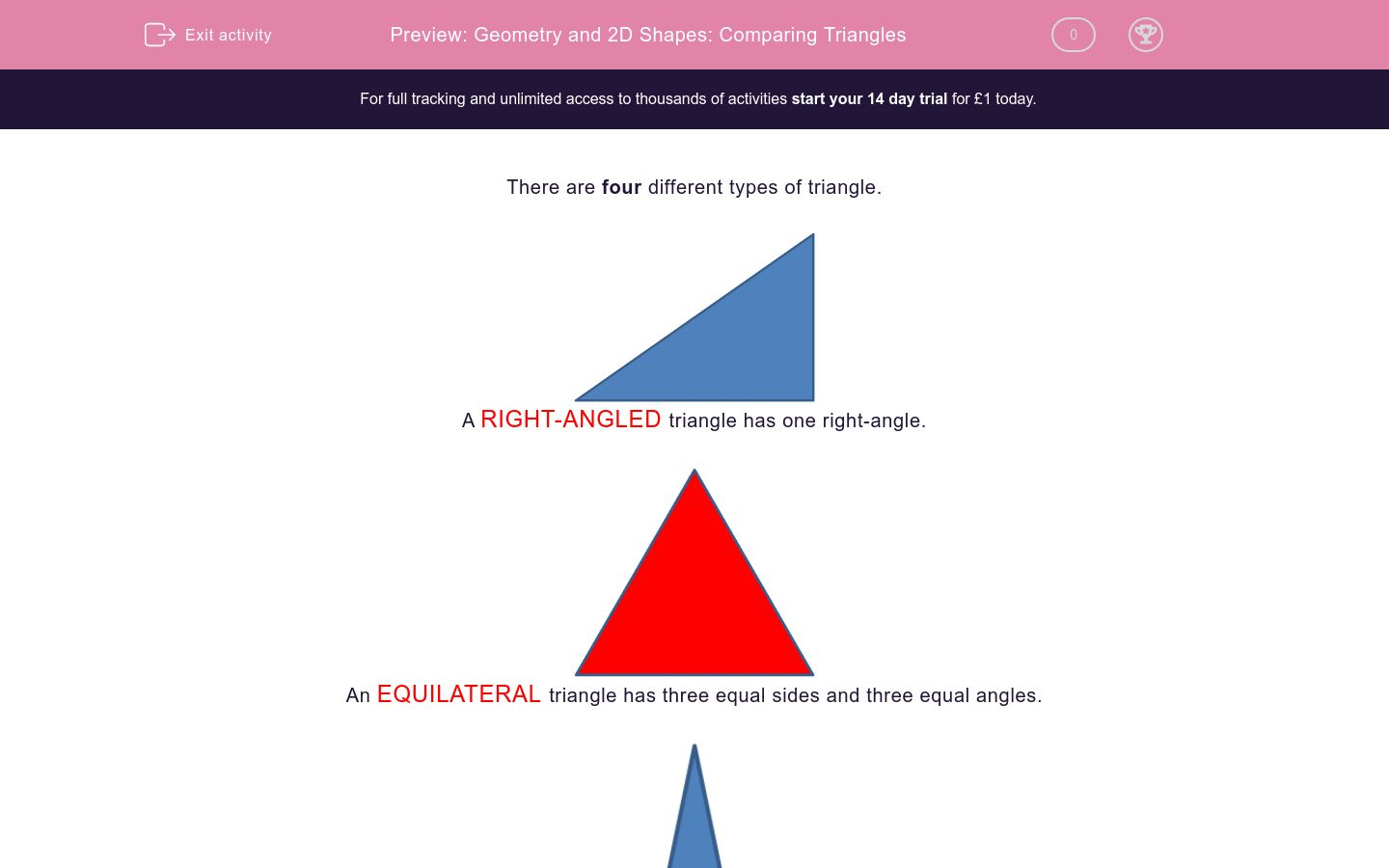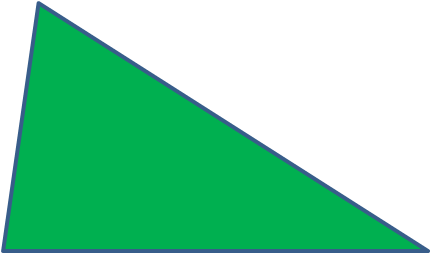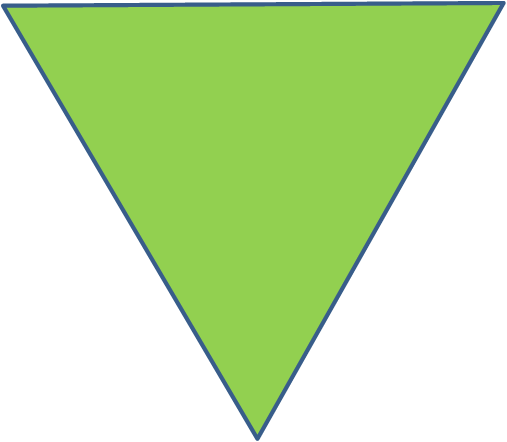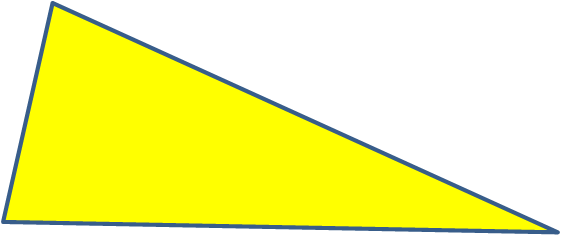# Geometry and 2D Shapes: Comparing Triangles

In this worksheet, students identify right-angled, isosceles, equilateral and scalene triangles.Key stage:  KS 2

Curriculum topic:   Maths and Numerical Reasoning

Curriculum subtopic:   2D Shapes: Triangles, Quadrilaterals and Polygons

Difficulty level:### QUESTION 1 of 10

There are four different types of triangle.RIGHT-ANGLED triangle has one right-angle.An EQUILATERAL triangle has three equal sides and three equal angles.An ISOSCELES triangle has two equal sides and two equal angles.SCALENE triangle has three different sides and three different angles.

Which sort of triangle is this?Equilateral

Isosceles

Right-Angled

Scalene

Which sort of triangle is this?Equilateral

Isosceles

Right-Angled

Scalene

Which sort of triangle is this?Equilateral

Isosceles

Right-Angled

Scalene

Which sort of triangle is this?Equilateral

Isosceles

Right-Angled

Scalene

Which sort of triangle is this?Equilateral

Isosceles

Right-Angled

Scalene

Which sort of triangle is this?Equilateral

Isosceles

Right-Angled

Scalene

Which sort of triangle is this?Equilateral

Isosceles

Right-Angled

Scalene

Which sort of triangle is this?Equilateral

Isosceles

Right-Angled

Scalene

Which sort of triangle is this?Equilateral

Isosceles

Right-Angled

Scalene

Which sort of triangle is this?Equilateral

Isosceles

Right-Angled

Scalene

• Question 1

Which sort of triangle is this?Isosceles
• Question 2

Which sort of triangle is this?Scalene
• Question 3

Which sort of triangle is this?Right-Angled
• Question 4

Which sort of triangle is this?Equilateral
• Question 5

Which sort of triangle is this?Scalene
• Question 6

Which sort of triangle is this?Equilateral
• Question 7

Which sort of triangle is this?Isosceles
• Question 8

Which sort of triangle is this?Right-Angled
• Question 9

Which sort of triangle is this?Scalene
• Question 10

Which sort of triangle is this?Right-Angled
---- OR ----

Sign up for a £1 trial so you can track and measure your child's progress on this activity.

### What is EdPlace?

We're your National Curriculum aligned online education content provider helping each child succeed in English, maths and science from year 1 to GCSE. With an EdPlace account you’ll be able to track and measure progress, helping each child achieve their best. We build confidence and attainment by personalising each child’s learning at a level that suits them.

Get started